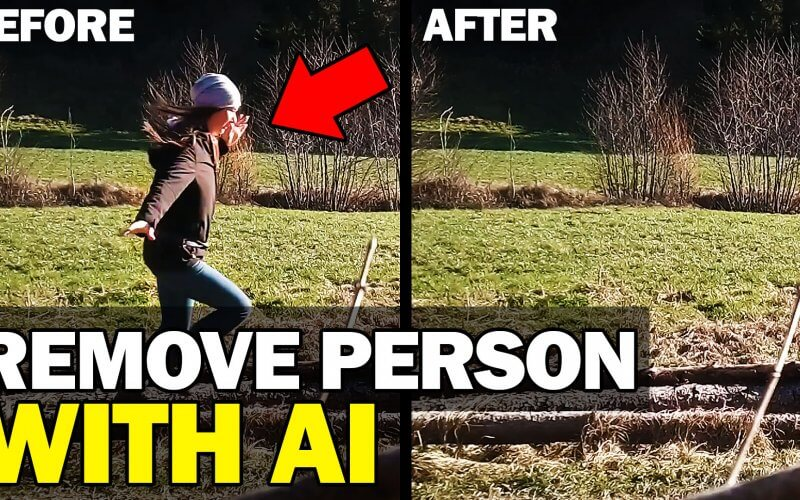# Remove Person from Video with AI and JavaScript

In this tutorial, we’re going to show you how to use Tensorflow.js and a pre-trained human segmentation machine learning model BodyPix to real-timely remove person from video (This tutorial is inspired by this project from Jason Mayes but the code is rewritten from scratch)

## Basic Setup

First let’s add video tag to our html page. I’ll set the video attributes to muted, loop and controls. We also need to create output canvas so we can display the processed video.

```<video id="video" width="800" src="test4.mp4" muted loop controls></video>
<canvas id="output-canvas" width="800" height="450"></canvas>```

Next I’m going to create an initialize function to get our video element (which will be used later) and our output canvas 2d context. Also create another canvas as a temporary working space. We will use this canvas to capture each video frame and image data, then process and put it into the output canvas for display.

Finally, we’ll start playing the video and call our new function “computeFrame” to start the processing.

```function init() {
video = document.getElementById("video");

c_out = document.getElementById("output-canvas");
ctx_out = c_out.getContext("2d");

c_tmp = document.createElement("canvas");
c_tmp.setAttribute("width",800);
c_tmp.setAttribute("height",450);
ctx_tmp = c_tmp.getContext("2d");

video.play();
computeFrame();
}```

## ML Model Setup

We’ll setup tensorflow.js and BodyPix using CDN.

```<script src="https://cdn.jsdelivr.net/npm/@tensorflow/tfjs/dist/tf.min.js"></script>
<script src="https://cdn.jsdelivr.net/npm/@tensorflow-models/[email protected]"></script>```

Next setup the model and segmentation configuration.

First is the neural network architecture. BodyPix supports both MobileNetV1 and ResNet50. In this tutorial, we’re going to use MobileNet which is faster but has less accuracy. Next are outputStride, multiplier and quantBytes. The higher number for these setting, the more segmentation accuracy at the cost of processing speed. (You can find more detail about each setting on this page)

```const bodyPixConfig = {
architechture: 'MobileNetV1',
outputStride: 16,
multiplier: 1,
quantBytes: 4
};```

Next is segmentation configuration. The first setting is internalResolution which you can choose to downsize the input image before process it to increase performance. In this tutorial, I’ll set it to “full” which means no resizing. segmentationThreshold is the minimum confident threshold before each pixel is considered part of human body. I’ll set it to 0.01 which means accept anything higher than 1% confident. The last one is scoreThreshold which is the minimum confident threshold to recognize the entire human body. Again I’ll set it to 0.01 (see image from Tensorflow blog below on how threshold works)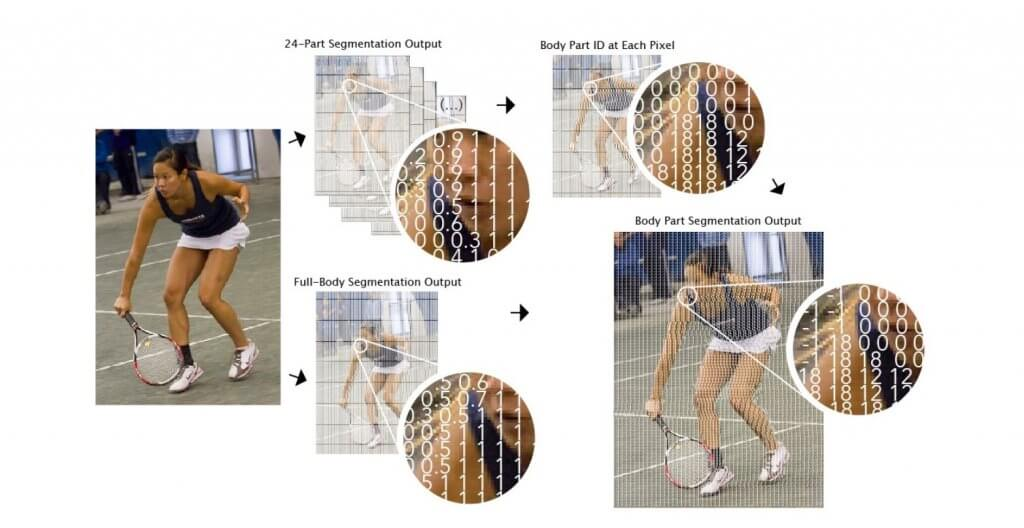```const segmentationConfig = {
internalResolution: 'high',
segmentationThreshold: 0.05,
scoreThreshold: 0.05
};```

Then I’m going to load the BodyPix model with our configuration.

```document.addEventListener("DOMContentLoaded", () => {
model = m;
init();
});
});```

Now back to our computeFrame function. First we’ll use the temp canvas and draw the current video frame on it using drawImage method. Then get the pixel data on the canvas using getImagedata.

After that we’ll call segmentPerson method using current image data on the canvas and our configuration to start analyzing. The result will be returned in a promise.

```function computeFrame() {
ctx_tmp.drawImage(video,0,0,video.videoWidth,video.videoHeight);
let frame = ctx_tmp.getImageData(0,0,video.videoWidth,video.videoHeight);

model.segmentPerson(c_tmp,segmentationConfig).then((segmentation) => {
//frame processing
});
}```

## Removing Human From Video

After the segmentation is completed, we’ll create a nested loop to go iterate through every pixels in the image. Since the image data is in single dimension array format. I’m going to create an index variable. “n” to access each pixel.

```model.segmentPerson(c_tmp,segmentationConfig).then((segmentation) => {
let out_image = ctx_out.getImageData(0,0,video.videoWidth,video.videoHeight);

for(let x=0;x<video.videoWidth;x++){
for(y=0;y<video.videoHeight;y++) {
let n = x + (y * video.videoWidth);
....```

Now here is the segmentation result from the model. It’s a single dimension array contains only 0 and 1. All non-human pixels will have zero value (Image from Tensorflow.js blog)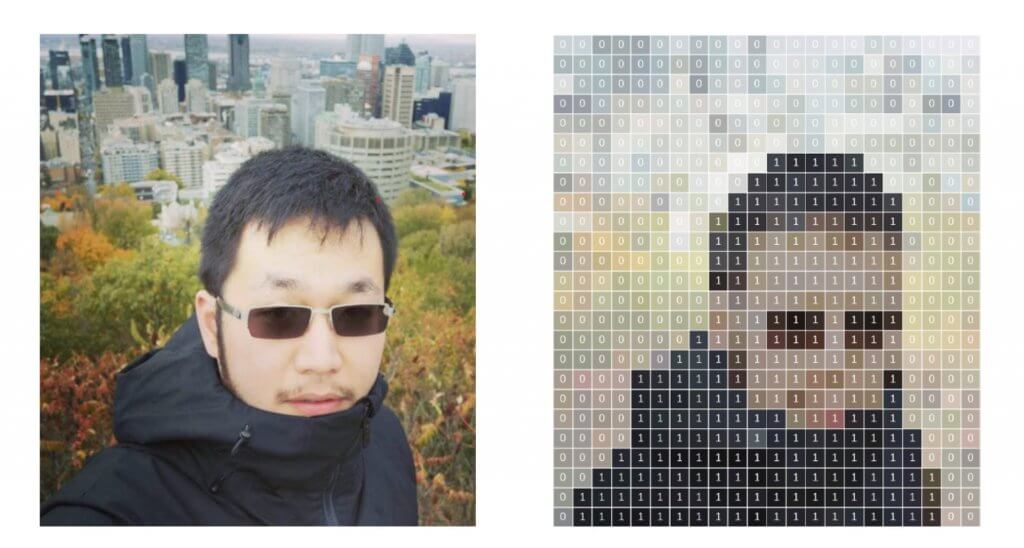So I’m going to create an if statement to check if the current pixel is NOT part of human, then we’ll copy the pixel data from the video to the output canvas. If it’s human, we’ll skip the updating process.
With this simple trick, we can remove the person from the video.

```if(segmentation.data[n] == 0) {
out_image.data[n * 4] = frame.data[n * 4];         //R
out_image.data[n * 4 + 1] = frame.data[n * 4 + 1]; //G
out_image.data[n * 4 + 2] = frame.data[n * 4 + 2]; //B
out_image.data[n * 4 + 3] = frame.data[n * 4 + 3]; //A
}```

You’ll notice that I multiply the index by 4. This because the each pixel has 4 datas, RGB and alpha which requires 4 array spaces for a single pixel (see images below)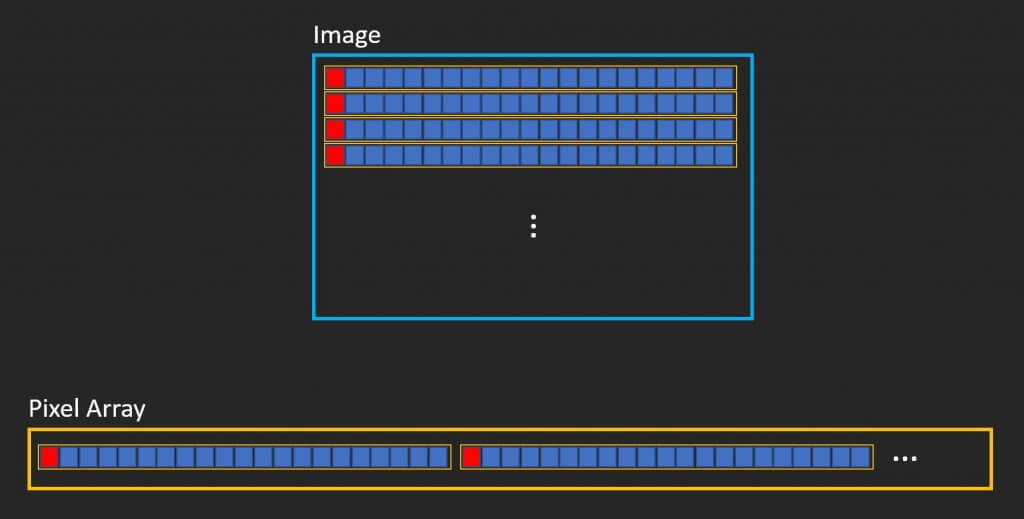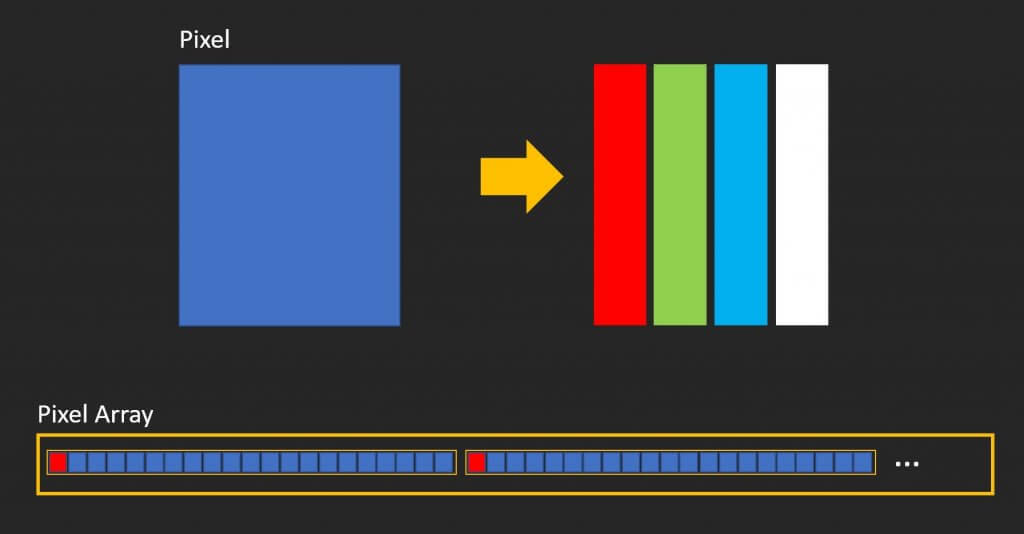The final step is to put the result to the output canvas. Also move the update inside the promise. And here is the final computeFrame function looks like

```function computeFrame() {
ctx_tmp.drawImage(video,0,0,video.videoWidth,video.videoHeight);
let frame = ctx_tmp.getImageData(0,0,video.videoWidth,video.videoHeight);

model.segmentPerson(c_tmp,segmentationConfig).then((segmentation) => {
let out_image = ctx_out.getImageData(0,0,video.videoWidth,video.videoHeight);

for(let x=0;x<video.videoWidth;x++){
for(y=0;y<video.videoHeight;y++) {
let n = x + (y * video.videoWidth);

if(segmentation.data[n] == 0) {
out_image.data[n * 4] = frame.data[n * 4]; //R
out_image.data[n * 4 + 1] = frame.data[n * 4 + 1]; //G
out_image.data[n * 4 + 2] = frame.data[n * 4 + 2]; //B
out_image.data[n * 4 + 3] = frame.data[n * 4 + 3]; //A
}
}
}
ctx_out.putImageData(out_image,0,0);
setTimeout(computeFrame,0);
});
}```

And here is the result. Not perfect but still amazing!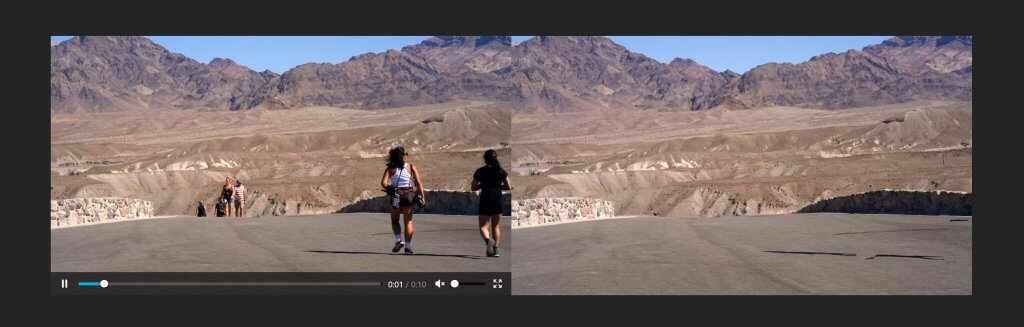You can download source code of this tutorial here or see the video below for demo.

## Take Away

You might have to readjust the model configuration for each video. Setting a low threshold will resulted in some non-human pixels being remove (see the branch at the bottom right)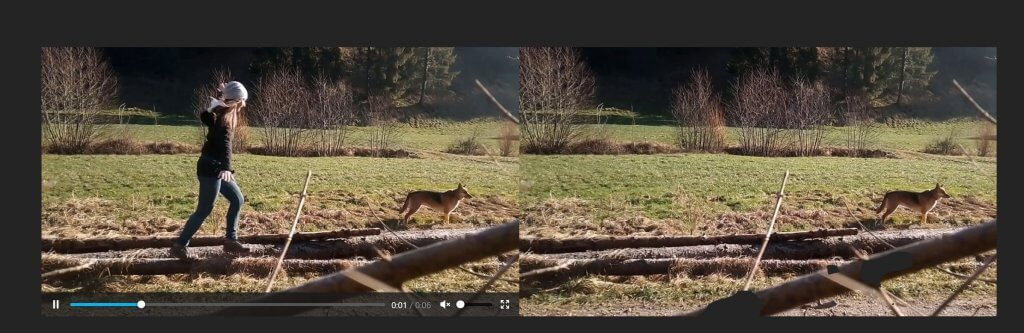Please also note that this trick have couple flaws. First if there is always human on a certain area of the video it won’t work. Also This technique requires fixed background video (No zooming/panning)

And that’s all for this tutorial. Hope you guys enjoy. If you want to see more development tips and tutorial videos, subscribe our channel to stay tune!

Written By
error: